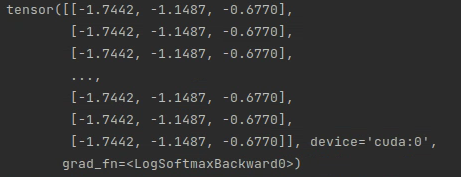# The error in Graph neural networks for node classification

I have several graphs and I use these graphs to train a model hoping to identify the category of each node.I have three categories, and here are the formulation of one of my graph(using the data object):

``````Data(x=[100,64],pos=[100,2],edge_index=[2,546],y=,edge_weight=)
``````

x is the embeding of every nodes, here have 100 nodes and each node have a 64-dimension feature, pos is the coordinate of each node. A two-dimensional vector in edge_index represents an edge between two points. And the edge_weight is the weight corresponding to each edge.

here is my GCN model:

``````import torch.nn as nn
import torch.nn.functional as F
from torch_geometric.nn import GCNConv

# GCN model with 2 layers
class Net(torch.nn.Module):
def __init__(self):
super(Net, self).__init__()
self.conv1 = GCNConv(data.num_features, 16)
self.conv2 = GCNConv(16, int(data.num_classes))

def forward(self):
x, edge_index = data.x, data.edge_index
x = F.relu(self.conv1(x, edge_index))
x = F.dropout(x, training=self.training)
x = self.conv2(x, edge_index)
return F.log_softmax(x, dim=1)

device = torch.device('cuda' if torch.cuda.is_available() else 'cpu')

data =  data.to(device)

model = Net().to(device)
``````

and here is my training code:

``````for epoch in range(epoches):
for img_path in img_paths: #in order to build the graph
data=build_graph(img_path)
model.train()